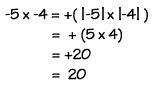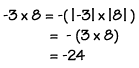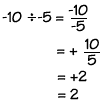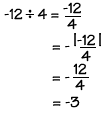Home    |    Teacher    |    Parents    |    Glossary    |    About UsYou multiply or divide integers just as you do whole numbers, except you must keep track of the signs. To multiply or divide signed integers, always multiply or divide the absolute values and use these rules to determine the sign of the answer.

When you multiply two integers with the same signs, the result is always positive. Just multiply the absolute values and make the answer positive.

Positive x positive = positive
Negative x negative = positive

When you multiply two integers with different signs, the result is always negative. Just multiply the absolute values and make the answer negative.

Positive x negative = negative
Negative x positive = negative

When you divide two integers with the same sign, the result is always positive. Just divide the absolute values and make the answer positive.

Positive ÷ positive = positive
Negative ÷ negative = positive

When you divide two integers with different signs, the result is always negative. Just divide the absolute values and make the answer negative.

Positive ÷ negative = negative
Negative ÷ positive = negative

Examples

1.2.3.4.Homework Help | Pre-Algebra | NumbersEmail this page to a friendSearch·  Place value·  Decimal numbers·  Estimating and     rounding·  Adding / subtracting     decimals·  Multiplying decimals·  Dividing decimals·  Percent·  Exponents·  Square roots·  Signed integers·  Adding and     subtracting integers·  Multiplying and     dividing integers·  Properties of integersFirst Glance In Depth Examples WorkoutMultiplying and Dividing Integers